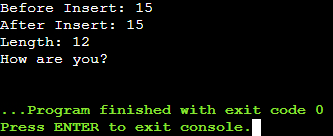# Append and Insert Functions of String Class in C++

## Append and Insert Functions of String Class in C++

In this article, I am going to discuss Append and Insert Functions of String Class in C++ Language with examples. Please read our previous article, where we discussed the Basic Functions of the String Class in C++ with examples.

##### Append and Insert Functions of String Class in C++

In this article, we will understand various member functions of the C++ String class. Already we have seen some basic functions in our previous article. We will explain the following functions one by one.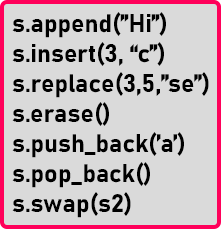##### append() Function of String Class in C++:

The first function is append. This function will add new content to a string. Suppose we have a string,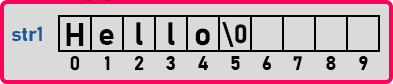In this string, we want to add some new words or characters. Then we will use the append function as “str1.append(“Roy”)”.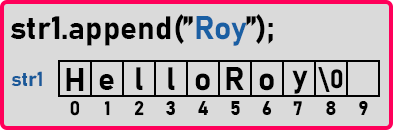Then “Roy” will be appended to this string and the string will become “HelloRoy”. Already we have seen that we have a string then the capacity is more than the size. In this string, we have more spaces also, so now the word “Roy” will be stored in that vacant spaces that are three spaces after this ‘Hello’ and if it requires it may increase the size. Actually, it will not increase the same array size, it will create a bigger size array and it will store it there.

So can’t say how this function works. This append function will handle. How it is defined inside the compiler we can’t see that. Let us see this function in the program.

##### Program for append function in C++:
```#include <iostream>
#include <string>
using namespace std;
int main()
{
string str = "Hello";
cout << "Before appending: " << str.capacity () << endl;
str.append ("Roy");
cout << "After appending: " << str.capacity () << endl;
cout << str << endl;
}
```
###### Output: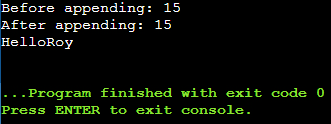You can see that capacity before append and after append is the same as we have not appended a large string. Now let’s see what happens if we append a large word or string.

##### Program for appending a large string using append function in C++:
```#include <iostream>
#include <string>
using namespace std;
int main()
{
string str = "Hello";
cout << "Before appending: " << str.capacity () << endl;
str.append (" Roy! How are you?");
cout << "After appending: " << str.capacity () << endl;
cout << "Length: " << str.length () << endl;
cout << str << endl;
}
```
###### Output: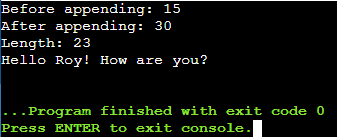As we have given a larger string so the capacity of the string is increased. Now let us continue with the next function i.e. Insert.

##### insert() Function of String Class in C++:

This function will insert a given string at a given index. Suppose we have a string,In this string, we want to insert “Roy” at index ‘0’ onwards. So, we will write, insert(0, “Roy”).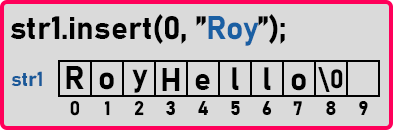Here “Roy” is inserted at the ‘0’ index. We can insert it at any location. Insert function takes 2 parameters, first is the index at where we want to insert in a string and second is the word or string which we want to insert.

There is another version of the insert function that is “insert(0, “Apple”, 2)”. This is taking 3 parameters. The first 2 parameters are the same but the last one is the total character we want to insert from the given word or string like “Apple”, so it will insert starting 2 characters from the word “Apple”. Now let us see this in the program.

##### Program for insert function in C++:
```#include <iostream>
#include <string>
using namespace std;
int main()
{
string str = "Hello";
cout << "Before Insert: " << str.capacity () << endl;
str.insert (0, "Roy ");
str.insert (9, ", How are you?");
cout << "After Insert: " << str.capacity () << endl;
cout << "Length: " << str.length () << endl;
cout << str << endl;
}
```
###### Output: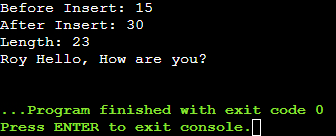Now let us see another version of the insert.

###### Program:
```#include <iostream>
#include <string>
using namespace std;
int main()
{
string str = "How you?";
cout << "Before Insert: " << str.capacity () << endl;
str.insert (3, " area", 4);
cout << "After Insert: " << str.capacity () << endl;
cout << "Length: " << str.length () << endl;
cout << str << endl;
}
```
###### Output: